## MP Board Class 6th Maths Solutions Chapter 11 Algebra Ex 11.5

Question 1.
State which of the following are equations (with a variable). Give reason for your answer. Identify the variable from the equations with a variable
(a) 17 = x + 7
(b) (t – 7) > 5
(c) $$\frac{4}{2}$$ = 2
(d) (7 × 3) – 19 = 8
(e) 5 × 4 – 8 = 2x
(f) x – 2 = 0
(g) 2m < 30
(h) 2n + 1 = 11
(i) 7 = (11 × 5) – (12 × 4)
(j) 7 = (11 ×2) + p
(k) 20 = 5y
(l) $$\frac{3q}{2}$$ < 5
(m) z + 12 > 24
(n) 20 – (10 – 5) = 3× 5
(o) 7 – x = 5
Solution:
(a) 17 = x + 7 is an equation with variable as both the sides are equal. The variable is x.
(b) (t – 7) > 5 is not an equation as L.H.S. is greater than R.H.S.
(c) $$\frac{4}{2}$$ = 2 is not an equation with variable.
(d) (7 × 3) – 19 = 8 is not an equation with variable.
(e) 5 × 4 – 8 = 2x is an equation with variable as both the sides are equal. The variable is x.
(f) x – 2 = 0 is an equation with variable as both the sides are equal. The variable is
(g) 2m < 30 is not an equation as L.H.S. is less than R.H.S.
(h) 2n + 1 = 11 is an equation with variable as both the sides are equal. The variable is n.
(i) 7 = (11 × 5) – (12 × 4) is not an equation with variable.
(j) 7 = (11 × 2) + p is an equation with variable as both the sides are equal. The variable is p.
(k) 20 = 5y is an equation with variable as both the sides are equal. The variable is
(l) $$\frac{3q}{2}$$ < 5 is not an equation as L.H.S. is less than R.H.S.
(m) z + 12 > 24 is not an equation as L.H.S. is greater than R.H.S.
(n) 20 – (10 – 5) = 3 × 5 is not an equation with variable.
(o) 7 – x = 5 is an equation with variable as both the sides are equal. The variable is x.

Question 2.
Complete the entries in the third column of the table.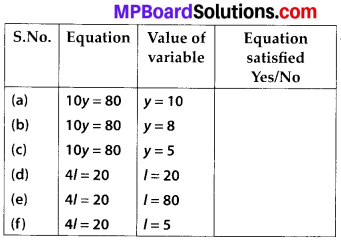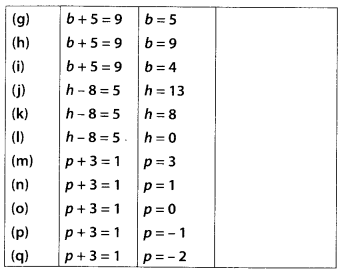Solution: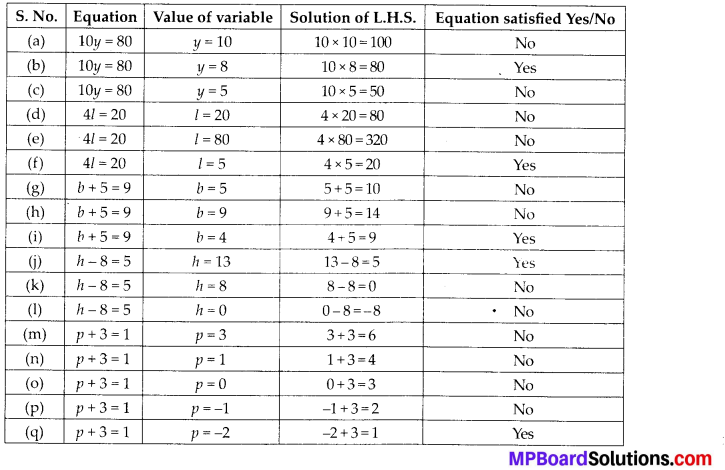Question 3.
Pick out the solution from the values given in the bracket next to each equation. Show that the other values do not satisfy the equation.
(a) 5m = 60        (10, 5, 12, 15)
(b) n + 12 = 20      (12, 8, 20, 0)
(c) p – 5 = 5       (0, 10, 5, -5)
(d) $$\frac{q}{2}$$ = 7   (7, 2, 10, 14)
(e) r – 4 = 0     (4, -4, 8, 0)
(f) x + 4 = 2      (-2, 0, 2, 4)
Solution:
(a) 5m = 60
Putting the given values in L.H.S., we get
5 × 10 = 50
∵ L.H.S. ≠ R.H.S.
∴ m = 10 is not the solution.
5 × 5 = 25
∵ L.H.S. ≠ R.H.S.
∴ m = 5 is not the solution.
5 × 12 = 60
∵ L.H.S. = R.H.S.
∴ m = 12 is a solution.
5 x 15 = 75
∵ L.H.S. ≠ R.H.S.
∴ m = 15 is not the solution.

(b) n + 12 = 20
Putting the given values in L.H.S., we get
12 + 12 = 24
∵ L.H.S. ≠ R.H.S.
∴ n = 12 is not the solution.
8 + 12 = 20
∵ L.H.S. = R.H.S.
∴ n = 8 is a solution.
20 + 12 = 32
∵ L.H.S. ≠ R.H.S.
∴ n = 20 is not the solution.
0 + 12 = 12
∵ L.H.S. ≠ R.H.S.
∴ n = 0 is not the solution.

(c) p – 5 = 5
Putting the given values in L.H.S., we get
0 – 5 = -5
∵ L.H.S. ≠ R.H.S.
∴ p = 0 is not the solution.
10 – 5 = 5
∵ L.H.S. = R.H.S.
∴ p = 10 is a solution.
5 – 5 = 0
∵ L.H.S. ≠ R.H.S.
∴ p = 5 is not the solution.
-5 – 5 = -10
∵ L.H.S. ≠ R.H.S.
∴ p = -5 is not the solution.

(d) $$\frac{q}{2}$$ = 7
Putting the given values in L.H.S., we get
$$\frac{7}{2}$$
∵ L.H.S. ≠ R.H.S.
∴ q = 7 is not the solution.
$$\frac{2}{2}$$ = 1
∵ L.H.S. ≠ R.H.S.
∴ q = 2 is not the solution.
$$\frac{10}{2}$$ = 5
∵ L.H.S. ≠ R.H.S.
∴ q = 10 is not the solution.
$$\frac{14}{2}$$ = 7
∵ L.H.S. = R.H.S.
∴ q = 14 is a solution.

(e) r – 4 = 0
Putting the given values in L.H.S., we get
4 – 4 = 0
∵ L.H.S. = R.H.S.
∴ r = 4 is a solution.
-4 – 4 = -8
∵ L.H.S. ≠ R.H.S.
∴ r = -4 is not the solution.
8 – 4 = 4
∵ L.H.S. ≠ R.H.S.
∴ r = 8 is not the solution.
0 – 4 = -4
∵ L.H.S. ≠ R.H.S.
∴ r = 0 is not the solution.

(f) x + 4 = 2
Putting the given values in L.H.S., we get
-2 + 4 = 2
∵ L.H.S. = R.H.S.
∴ x = -2 is a solution.
0 + 4 = 4
∵ L.H.S. ≠ R.H.S.
∴ x = 0 is not the solution.
2 + 4 = 6
∵ L.H.S. ≠ R.H.S.
∴ x = 2 is not the solution.
4 + 4 = 8
∵ L.H.S. ≠ R.H.S.
∴ x = 4 is not the solution.Question 4.
(a) Complete the table and by inspection of the table, find the solution to the equation m + 10 = 16.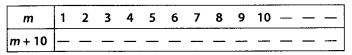(b) Complete the table and by inspection of the table, find the solution to the equation 5t = 35.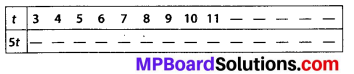(c) Complete the table and find the solution of the equation z/3 = 4 using the table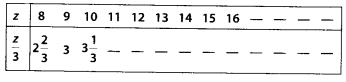(d) Complete the table and find the solution to the equation m – 7 = 3.Solution: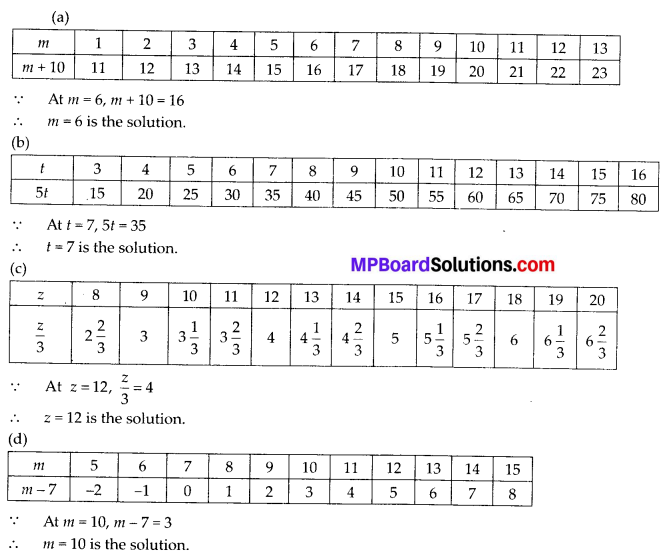Question 5.
Solve the following riddles, you may yourself construct such riddles.
Who am I?
(i) Go round a square
Counting every corner
Thrice and no more!
To get exactly thirty four!

(ii) For each day of the week
Make an upcount from me
If you make no mistake
You will get twenty three!

(iii) I am a special number
Take away from me a six!
A whole cricket team
You will still be able to fix!

(iv) Tell me who I am
I shall give a pretty clue!
You will get me back
If you take me out of twenty two!
Solution:
(i) According to given information, we have
3(4) + x = 34 ⇒ 12 + x = 34
⇒ x = 34 – 12 ⇒ x = 22(ii) According to given information, we have
x + 7 = 23
⇒ x = 23 – 7 ⇒ x = 16

(iii) According to given information, we have
x – 6 = 11
⇒ x = 11 + 6 ⇒ x = 17 .

(iv) According to given information, we have
x = 22-x ⇒ x + x = 22 ⇒ 2x = 22
⇒ x = $$\frac{22}{2}$$ ⇒ x = 11